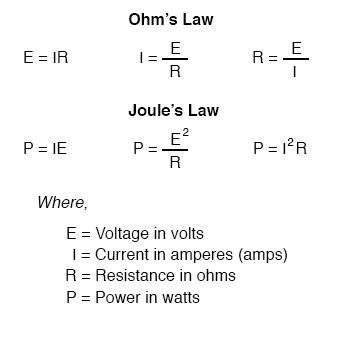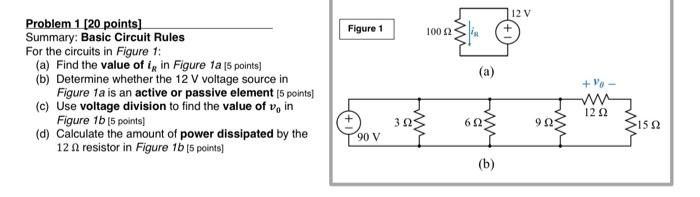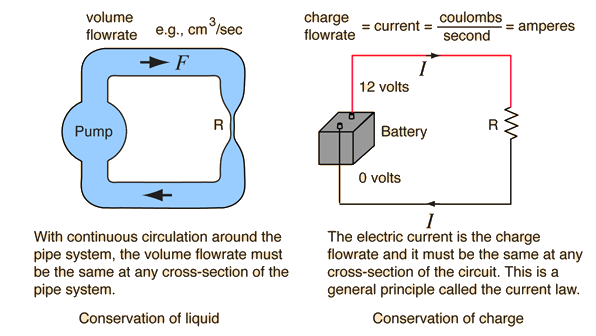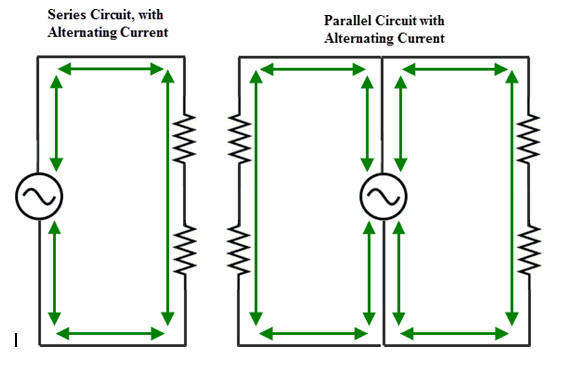# Basic Circuit Rules

By | March 3, 2023

As technology continues to evolve and become increasingly complex, there is a critical need for individuals to understand the basics of circuitry in order to develop a working knowledge of how electrical systems operate.

For those who are new to the field, the concept of electricity and its associated rules can be a bit daunting. Fortunately, basic circuit rules provide a simple foundation that can be used to better understand and build upon the fundamentals of electricity.

Let's start with the most basic of all circuit rules -- Ohm's law. This law states that the current in a circuit is directly proportional to the voltage and inversely proportional to the resistance. In other words, if the voltage increases, the current will increase, and if the resistance increases, then the current will decrease. In addition to this, Ohm's law also tells us that the power dissipated in a circuit is equal to the voltage multiplied by the current.

The next basic circuit rule is Kirchhoff's current law, which states that the sum of all currents entering any point in a circuit must equal the sum of all currents leaving the same point. This law is vital for determining the direction of current flow in a circuit.

Kirchhoff's voltage law is also important for anyone studying electrical circuits. This law states that the sum of all voltages around any closed loop in a circuit is equal to zero. This law allows us to calculate the voltages at different points in a circuit by knowing the voltages and resistances of certain components in the circuit.

Finally, it's important to understand the concept of resistance in a circuit. Resistance is simply the opposition to the flow of current in a circuit. In electrical terms, this usually means that the higher the resistance, the lower the amount of current flowing through the circuit. This means that resistors are often used in circuits to control the amount of current flowing through them.

These basic circuit rules form the foundation of any electrical circuit, and understanding them is key to developing a working knowledge of electricity. By applying these principles, engineers and technicians are able to build and troubleshoot more complex electrical systems.

Whether you're a professional or a beginner in the field of electrical engineering, mastering the basics of circuit rules and understanding how electricity works is an essential part of the success. With a firm grasp on the fundamental principles of electricity, you'll be well on your way to becoming an expert in the field.Circuit Topology And Laws Applied ElectricitySeries And Parallel Circuits Sparkfun LearnBasic Electrical Theory Ohms Law Cur Circuits MoreDc Circuit Equations And Laws Useful Conversion Factors Electronics TextbookCircuit Calculations Series Circuits Basic Rules A Has Certain Characteristics And 1 The Same Cur Flows Through Each PptCircuit Calculations Series Circuits Basic Rules A Has Certain Characteristics And 1 The Same Cur Flows Through Each PptElectrical Electronic Series CircuitsResistance Physics Series And Parallel Resistor Circuits PptComplex Circuit Stickman PhysicsThe Difference Between Series And Parallel Circuits Basic Direct Cur Dc Theory Automation TextbookCircuit Analysis For Dummies Cheat SheetBasic Laws Of Electric Circuits Nodes Branches Loops And Ppt OnlineSeries And Parallel Circuits Sparkfun LearnOhms LawSolved 12 V Figure 1 10012 R A Problem 20 Points Chegg ComBasic Circuit LawsWater Circuit Analogy To ElectricBasic Electrical Theory Ohms Law Cur Circuits More